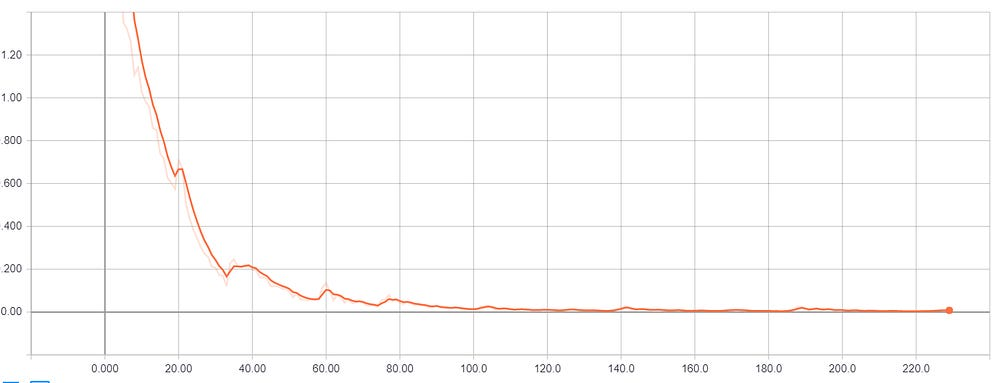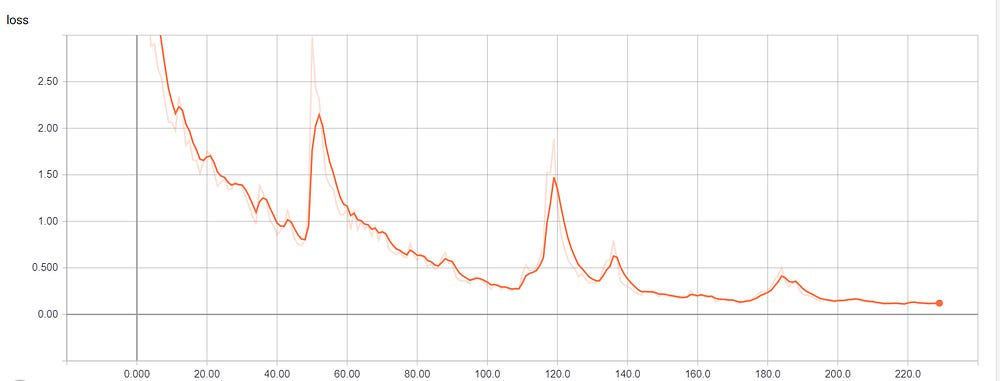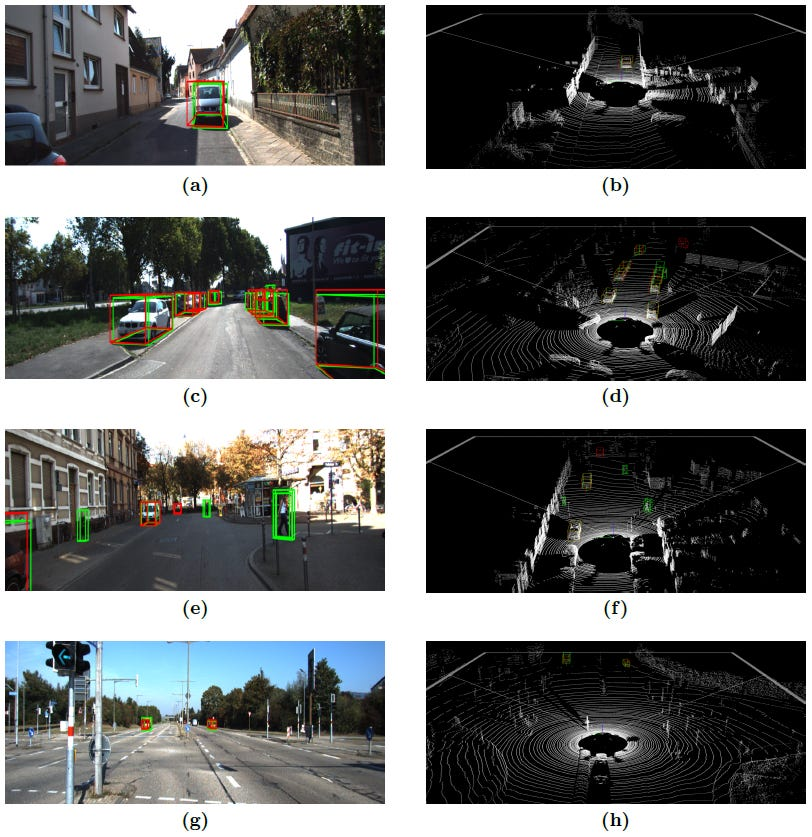# 3D Object Detection from LiDAR Data with Deep Learning

Source: Deep Learning on Medium

Author: Alex Nasli

Object detection is a key task in autonomous driving. The autonomous cars are usually equipped with multiple sensors such as camera, LiDAR. Although Convolutional Neural Networks are the state of the art techniques for 2D object detection, they do not perform well on 3D point cloud due to the sparse sensor data, therefore new techniques are needed. The 3D object detection networks work on the 3D point cloud provided by a range distance sensor. In this thesis, the LiDAR-based networks are detailed and implemented, like theVoxelNet. VoxelNet is an end-to-end network that combines feature extraction and bound-ing box prediction.VoxelNet is trained on KITTI car benchmark. The main performance evaluation metric is the mean average precision.

### VoxelNet overview

VoxelNet is an end-to-end network that combines feature extraction and bounding box prediction. This network works directly on 3D point cloud data. The network generates 3D bounding boxes from the point cloud, as shown in Figure 1.

First, the 3D space is divided into equally spaced voxels. The points are grouped according to the voxel they belong to.
The first layer of the VoxelNet is an encoding layer which transforms a group of points within each voxel into a feature representation, a 4D tensor. The layer is called Voxel Feature Encoding layer.
Then a 3D convolution is applied to the input tensor to aggregate voxel-wise features.
The output of the convolutional middle layer is the input of the RPN layer. The RPN produces a probability score map and a regression map. The RPN is shown in Figure 2. The loss is the sum of the classification loss and the regression loss.

#### Dataset

KITTI 3D object detection benchmark contains 7481 training samples and 7518 testing samples. The ground truth labels of the test set are not available, therefore, for the training, we will subdivide the 7481 training samples into a training set and a validation set. The training set contains 3712 data, and the validation set contains 3769 data. Each data includes the LiDAR points, the picture of the left camera, the corresponding projection matrices, and the labels.

#### Training

I implemented the VoxelNet in Keras. The Keras implementation is used for detecting cars only. Moreover, the hyperparameters are modified to have better experimental results. The network was trained on a single sample, and on a small dataset. An anchor box is considered positive if it has the highest IOU with any ground truth or the IOU is above 0.6 with any ground truth boxes. An anchor box is evaluated as negative if the IOU with all ground truth box is less than 0.45. Those anchor boxes are considered as don’t cares which
have their IOU value between 0.45 and 0.6. In  stochastic gradient descent was used with learning rate 0.01 for the first 150 epochs and 0.001 for the last 10 epoch. Instead of using stochastic gradient descent, the network was optimized with Adam optimizer with 0.001 learning rate, beta1 = 0:9, and beta2 = 0:999 for 230 epochs.

First, the VoxelNet was trained on a single sample to show an overfitting result. With these settings, the following training loss was produced as it is shown in Figure 3, which was plotted in TensorBoard. Next, the VoxelNet was trained on a small dataset with the batch size of 2. The next training loss was produced in Figure 4, which was plotted in TensorBoard.Figure 3. The training loss after training on a single sample.Figure 4. The training loss after training on a small dataset.

#### Prediction

First, the network was trained on a single sample. The network’s training loss value was decreased to close to zero. This can be tested with predicting on the training sample. The inference time of a prediction is around 0.7sec.

Second, the network was trained on a mini dataset. In Figure 5 some predictions are shown on the training samples. Certainly, the network predicted some false positive bounding boxes. In Figure 5, the green boxes represent the ground truth, and the red boxes represent the predicted boxes. In Figure 5, the scenes on the right side are plotted in 3D with the Mayavi Python library. The white points are the measurements from the LiDAR sensor.

#### Summary

In this part of my thesis work, I got familiar with 2D-3D object detections. Although Convolutional Neural Networks are the state of the art techniques for 2D object detection, they do not perform well on 3D point cloud due to the sparse sensor data. Therefore, some pre-operation is needed on the 3D point cloud.
VoxelNet divides the 3D space into voxels, transforms the voxels into a matrix representation which encodes the point interaction within a voxel. Convolutional Neural Networks extracts the complex features and outputs the confidence values and the regression values of the bounding boxes. I implemented the VoxelNet in Keras. I visualized the prediction on the images from the Kitti benchmark. I used Mayavi for plotting the 3D LiDAR points with the bounding boxes.Figure 5. Predictions on the training samples. White: the LiDAR pointcloud, green: the ground truth bounding boxes, red: the predicted bounding boxes. The 3D scene is plotted in Mayavi.

#### Bibliography

 Yin Zhou and Oncel Tuzel. Voxelnet: End-to-end learning for point cloud based 3dobject detection.arXiv preprint arXiv:1711.06396, 2017.